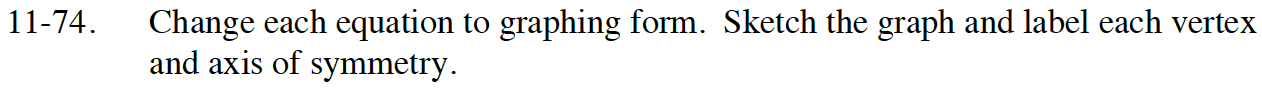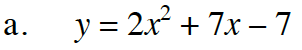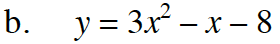Home > INT3 > Chapter 11 > Lesson 11.2.2 > Problem11-74

11-74.
1. Change each equation to graphing form. Sketch the graph and label each vertex and axis of symmetry. Homework Help ✎

1. y = 2x2 + 7x – 7

2. y = 3x2x – 8Add 7 to both sides to isolate the x-terms.

$y+7=2x^2+7x$

Factor out a 2 from the x-terms.

$y+7=2\left(x^2+\frac{7}{2}x \right)$

Complete the square. Remember to add to both sides.

$y+7+\frac{98}{16}=2\left( x^2+\frac{7}{2}x+\frac{49}{16} \right)$

Simplify.

$y+\frac{105}{8}=2\left( x+\frac{7}{4} \right)^2$

Where is the vertex? Axis of symmetry?See part (a).